## College Physics

Physics & Astronomy

## Quiz 2 : Electrical Energy and CapacitanceLooking for Introductory Physics Homework Help?# Quiz 2 : Electrical Energy and Capacitance

(a) The path followed by a positive test charge in uniform magnetic field gives the direction of the field. If the electric field is due to a positive charge, then the test charge will move away from the charge and hence the direction of the electric field will be away from the positive charge.. If the electric field is due to a negative charge, then the test charge will move towards the charge and hence the direction of the electric field will be towards the negative charge. Since the proton is a positively charged particle, it will move in the direction of the uniform electric field when it is released from rest. (b) The total energy of the proton in this uniform electric field is the sum of its kinetic energy and its potential energy and the total energy of the proton always remains constant. The energy possessed by a proton by virtue of its motion is called kinetic energy. The kinetic energy of the moving proton is given as follows: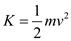Here, m is mass of the proton and v is the velocity of the proton. From the above equation it is clear that the kinetic energy of the proton is directly proportional to the square of the velocity of the proton. Thus, if the velocity of the proton increases, then the kinetic energy of the proton increases and hence the potential energy associated with the proton decreases.

(a) The electron is a negatively charged particle, thus it will move in the direction opposite to the direction of the uniform electric field when it is released from rest. Since the field is directed in positive-x direction, the electron moves in negative- x direction. Let q be the charge of the electron and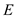be the strength of the electric field. So, the force experienced by the electron in the electric field is,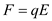The work done by the applied field to displace the electron through a distance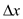is given as follows: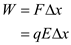Substitute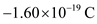for q ,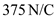for E , andfor.Therefore, the amount of work done by the electric field is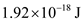. (b) The change in the electric potential energy is equal to the negative of the work done on the particle by the field. Thus, the change in potential energy of the electron is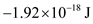(c) The law of conservation of energy states that in the absence of non-conservative forces, the total energy of the system remains constant. Since the electric forces are conservative forces the increase in kinetic energy of the electron is equal to decrease in potential energy of the electron. That is,Here, m is mass of the electron and v is the speed of the electron. Rearrange above equation for v.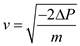Substitutefor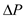and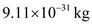for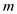.Therefore, the velocity of the electron is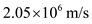and is directed in the negative x -direction.

(a) The electrostatic potential energy between a pair of point charges is directly proportional to the product of the magnitudes of the charges and inversely proportional to the distance between the charges.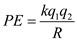Here, k is the Coulomb constant,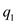and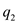are the magnitudes of the two charges and R is the distance between them. The potential energy between the point objects for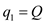,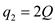, and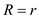is,(b) The potential energy between the point objects for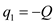,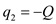andis,(c) The potential energy between the point objects for,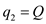and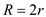is,(d) The potential energy between the point objects for,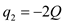andis,From the above calculated values, it is clear that potential energy in case a is greatest among the given cases and potential energy in case c is the smallest. The potential energy in case b and case d is same. Therefore, the potential energies of the given systems can be ranked as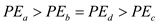.

There is no answer for this question